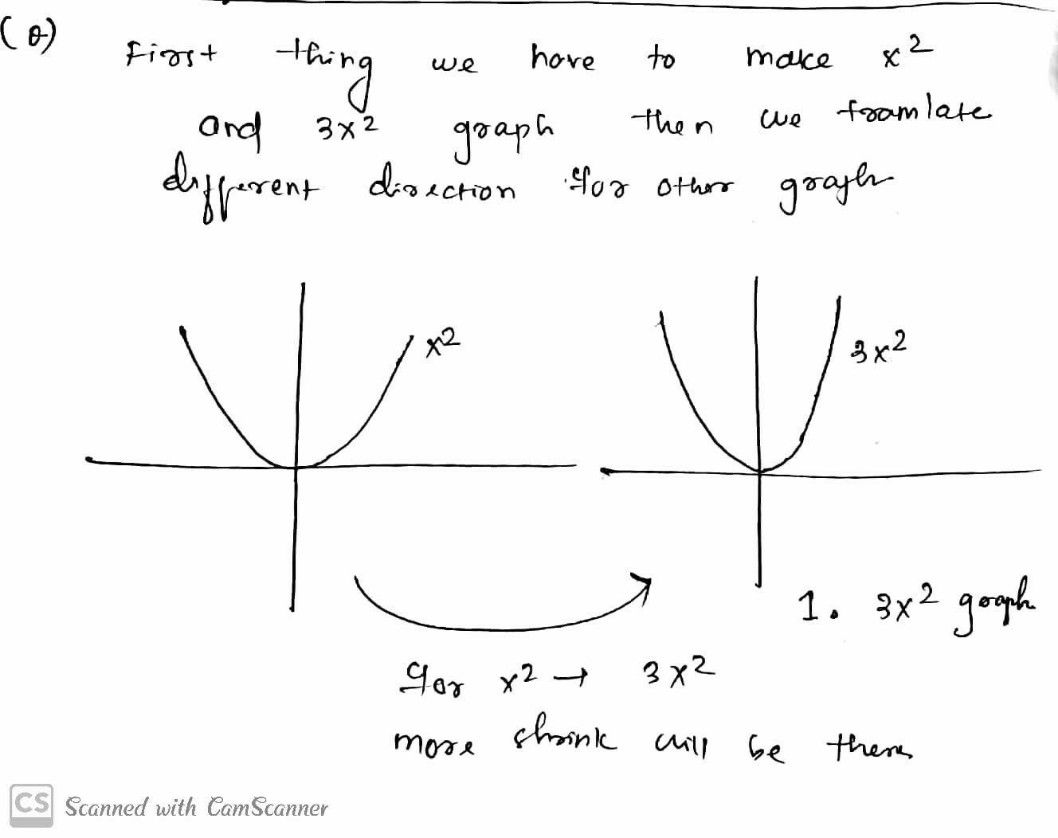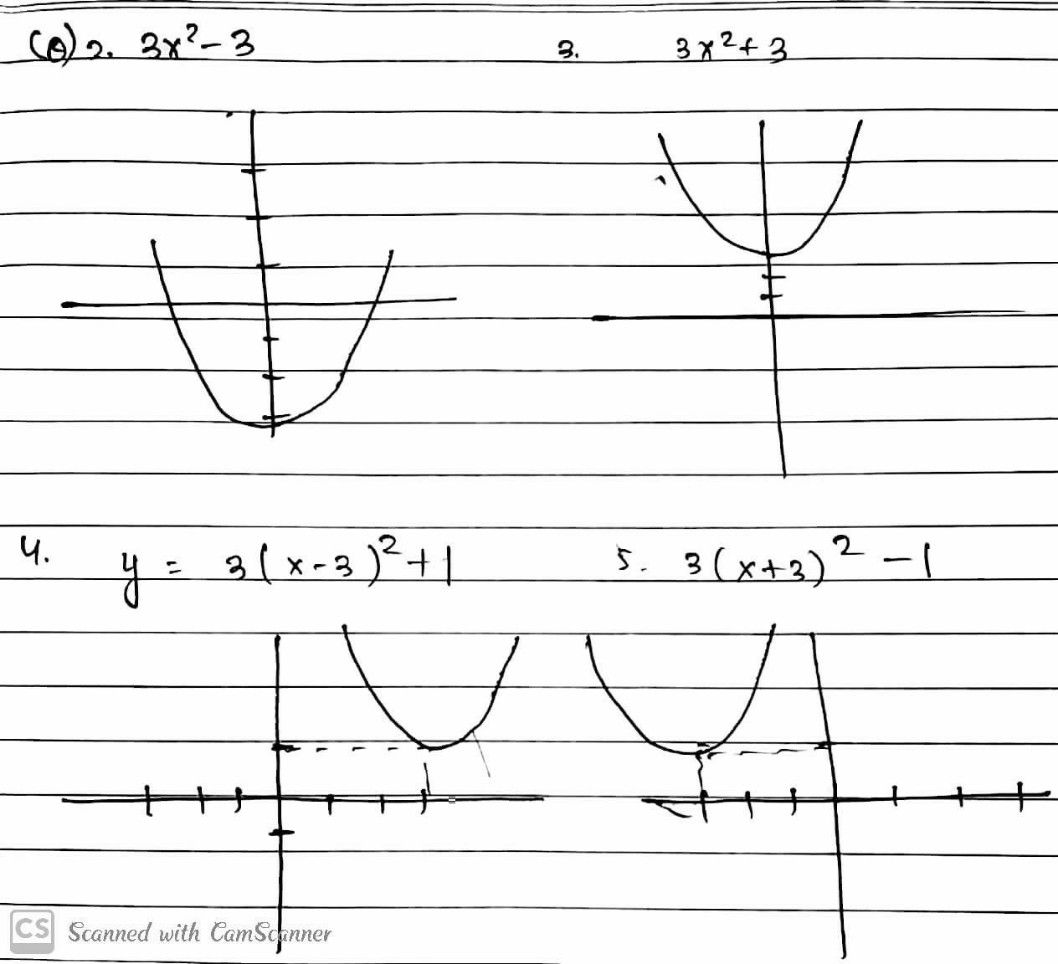Symbol
Problemzearning T'ask $4°$ Illustrate the graphs of the following quadratic functions then analyze the effects from each other. $1.y=3x^{2}$ 3 $2.y=3x^{2-3}$ $2$ $3.y=3x2+3$ 1 $4.y=3\left(x-3\right)^{2+1}$ -7 $6$ $\bar{5}$ $\bar{-3}$ $\bar{-2}$ -1 $1$ $\bar{2}$ $3$ $8$ $5.y=3\left(x+3\right)^{2-1}$ $-2$ $-3$ $Explanation:$
7th-9th grade
Other
Search count: 105
Question content
How to do this in a graph? What is the whole explanation here?
SolutionQanda teacher - RRmam